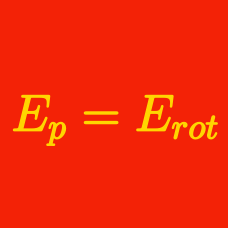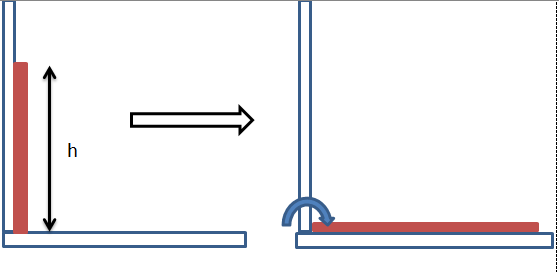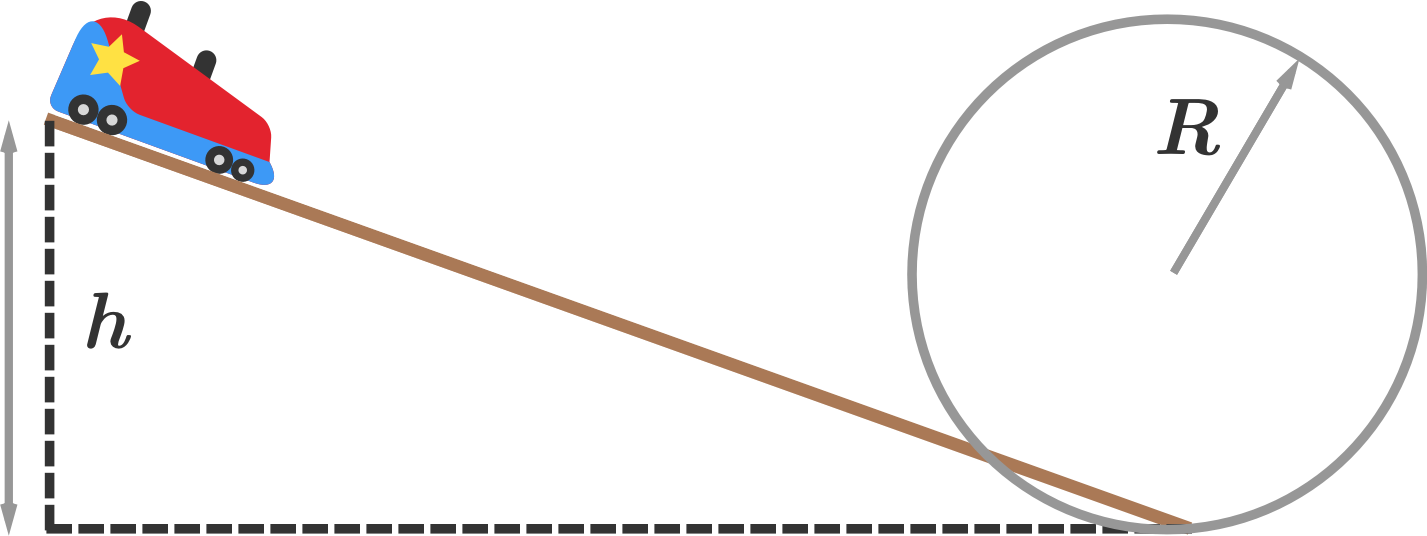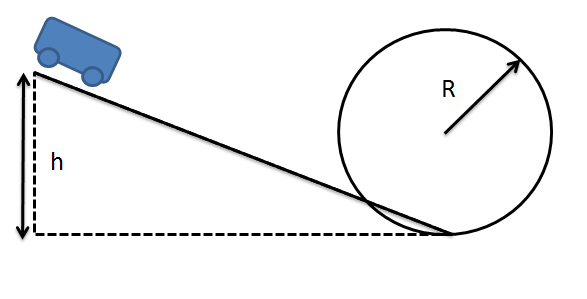Classical Mechanics

# Conservation of total mechanical energyA rod of mass $3\text{ kg}$ with homogeneous density stands against a vertical wall. The rod starts to fall clockwise, with the bottom of the rod fixed to the corner. If the length of the rod is $h=8 \text{ m},$ what is the squared velocity of the rod's center just before it hits the ground?

The gravitational acceleration is $g = 10 \text{ m/s}^2.$

An object initially at rest is dropped, and starts to free fall. When the object is $60 \text{ m}$ above the ground, its velocity is $10 \text{ m/s}.$ If the air resistance is negligible, from what height was the object dropped?

The gravitational acceleration is $g = 10 \text{ m/s}^2.$A rod of mass $2\text{ kg}$ with homogeneous density stands against a vertical wall. The rod starts to fall clockwise, with the bottom of the rod fixed to the corner. If the length of the rod is $h=3 \text{ m},$ what is the squared velocity of the end of the rod just before it hits the ground?

The gravitational acceleration is $g = 10 \text{ m/s}^2.$A roller-coaster initially at rest slides down a down-hill track, and then proceeds through a circular loop track of radius $R=6\text{ m}.$ When the roller-coaster is at the highest point of the loop, it is traveling at the minimum speed required to stay on the track and not fall down. If the whole track is perfectly frictionless, what was the initial height $h$ of the roller-coaster?

The gravitational acceleration is $g=10\text{ m/s}^2.$A roller-coaster initially at rest slides down a down-hill track, and then proceeds through a circular loop track. When the roller-coaster is at the highest point of the loop, it is traveling at the minimum speed required to stay on the track and not fall down. If the initial height of the roller-coaster was $h=50\text{ m},$ what is the radius $R$ of the loop?

The whole track is perfectly frictionless, and the gravitational acceleration is $g=10\text{ m/s}^2.$

×